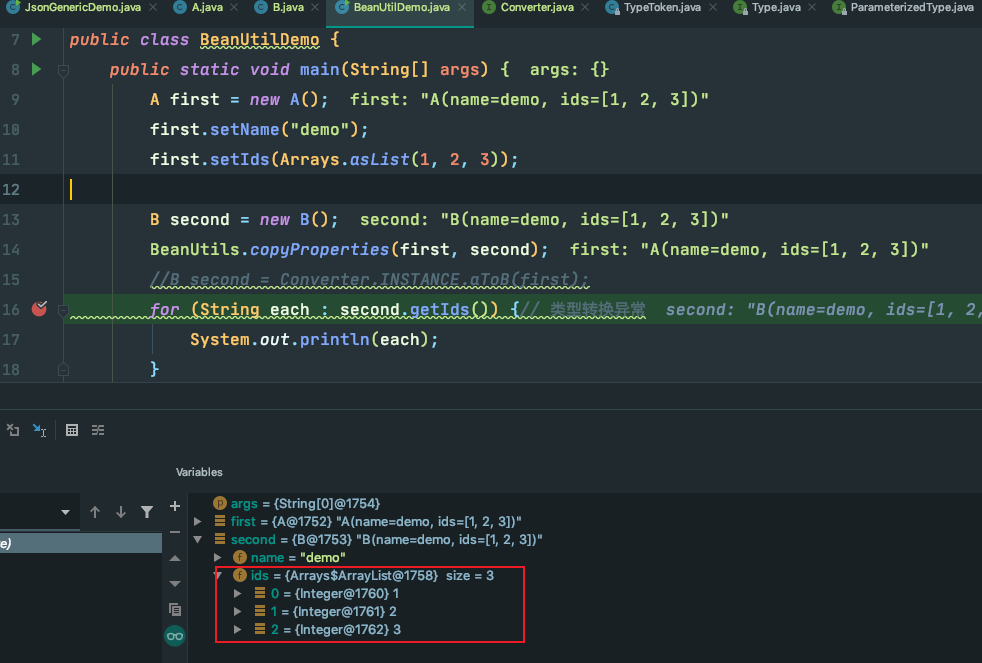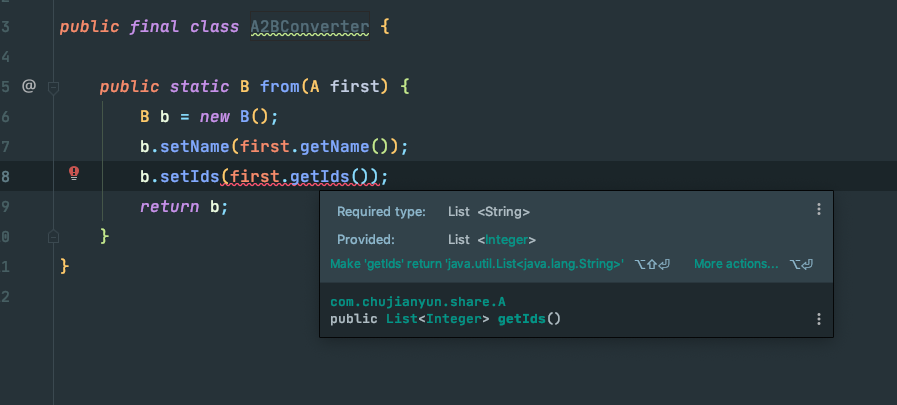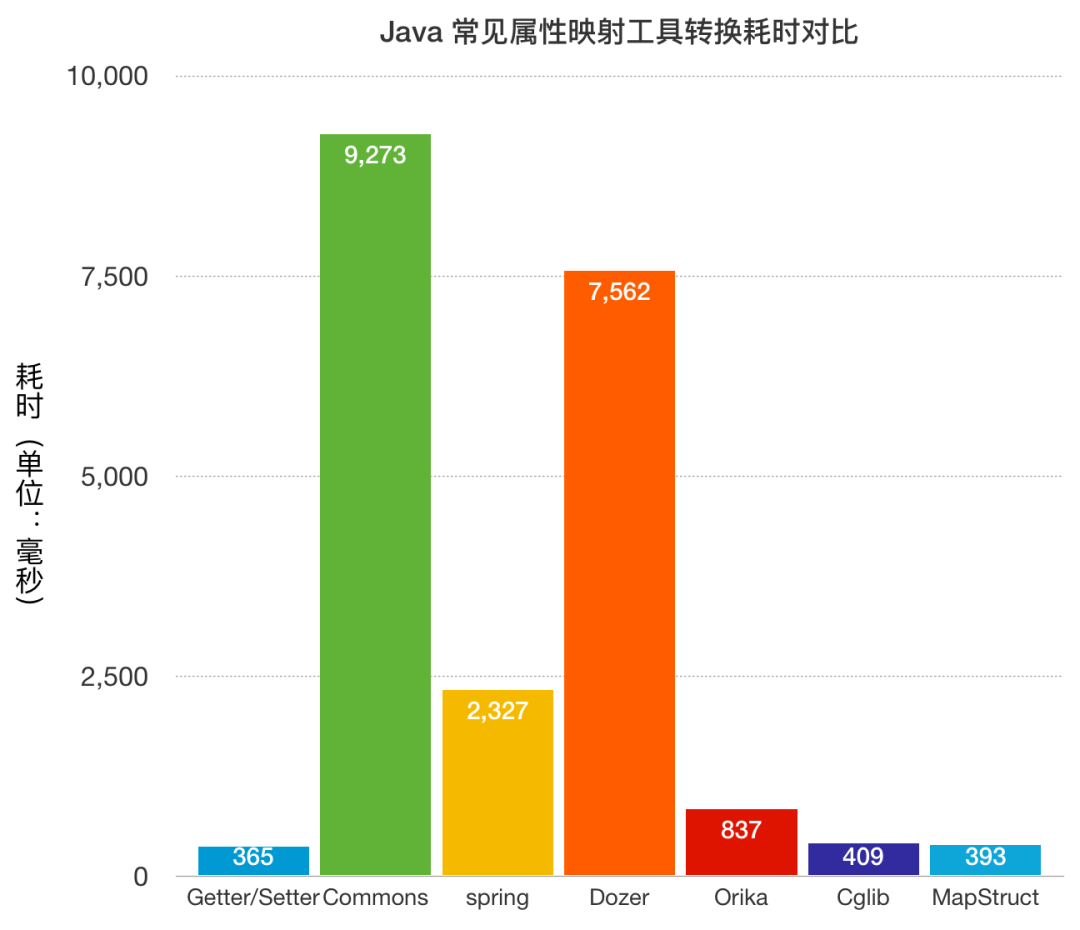# 为什么不推荐使用BeanUtils属性转换工具

1、【建议收藏】面试官会问的位运算奇淫技巧2、Top 16 的 Java 工具类，你用过几个？3、到底可不可以用 kill -9 关闭程序？4、IDEA 2021首个大版本发布，新增了这几个超实用功能！5、Optional 是个好东西，你真的会用么？


# 2、示例

import lombok.Data;
import java.util.List;

@Data
public class A {
private String name;

private List<Integer> ids;
}
@Data
public class B {
private String name;

private List<String> ids;
}
import org.springframework.beans.BeanUtils;

import java.util.Arrays;

public class BeanUtilDemo {
public static void main(String[] args) {
A first = new A();
first.setName("demo");
first.setIds(Arrays.asList(1, 2, 3));

B second = new B();
BeanUtils.copyProperties(first, second);
for (String each : second.getIds()) {// 类型转换异常
System.out.println(each);
}
}
}import org.easymock.cglib.beans.BeanCopier;

import java.util.Arrays;

public class BeanUtilDemo {
public static void main(String[] args) {
A first = new A();
first.setName("demo");
first.setIds(Arrays.asList(1, 2, 3));

B second = new B();
final BeanCopier beanCopier = BeanCopier.create(A.class, B.class, false);
beanCopier.copy(first,second,null);

for (String each : second.getIds()) {// 类型转换异常
System.out.println(each);
}
}
}



import org.mapstruct.Mapper;
import org.mapstruct.factory.Mappers;

@Mapper
public interface Converter {
Converter INSTANCE = Mappers.getMapper(Converter.class);

B aToB(A car);
}
import java.util.Arrays;

public class BeanUtilDemo {
public static void main(String[] args) {
A first = new A();
first.setName("demo");
first.setIds(Arrays.asList(1, 2, 3));

B second = Converter.INSTANCE.aToB(first);
for (String each : second.getIds()) {// 正常
System.out.println(each);
}
}
}



import java.util.ArrayList;
import java.util.List;
import javax.annotation.Generated;
import org.springframework.stereotype.Component;

@Generated(
value = "org.mapstruct.ap.MappingProcessor",
comments = "version: 1.3.1.Final, compiler: javac, environment: Java 1.8.0_202 (Oracle Corporation)"
)
@Component
public class ConverterImpl implements Converter {

@Override
public B aToB(A car) {
if ( car == null ) {
return null;
}

B b = new B();

b.setName( car.getName() );
b.setIds( integerListToStringList( car.getIds() ) );

return b;
}

protected List<String> integerListToStringList(List<Integer> list) {
if ( list == null ) {
return null;
}

List<String> list1 = new ArrayList<String>( list.size() );
for ( Integer integer : list ) {
}

return list1;
}
}



@Override
public B aToB(A car) {
if ( car == null ) {
return null;
}

B b = new B();

b.setName( car.getName() );
if ( car.getNumber() != null ) { // 问题出在这里
b.setNumber( Long.parseLong( car.getNumber() ) );
}
b.setIds( integerListToStringList( car.getIds() ) );

return b;
}



public final class A2BConverter {

public static B from(A first) {
B b = new B();
b.setName(first.getName());
b.setIds(first.getIds());
return b;
}
}3、结论

mapstruct 自定义了注解处理器，在编译阶段可以读取映射双方的泛型类型，进而进行映射。但是这种映射也很可怕，有时候我们由于粗心等原因定义错了类型，自动帮助我们进行了转换，会带了很多副作用。往期热门文章：

1、《历史文章分类导读列表！精选优秀博文都在这里了！》
2、七种方式教你在Spring Boot初始化时搞点事情
3、ConcurrentHashMap有十个提升性能的地方，你都知道吗？
4、程序员等级图鉴
5、Java 中的 Switch 都支持 String 了，为什么不支持 long？
6、为什么数据库字段要使用NOT NULL？

7、CTO 说了，用错 @Autowired 和 @Resource 的人可以领盒饭了
8、程序员离职事件始末

9、别总写代码，这130个网站比涨工资都重要
10、程序员养生指北


05-271205-0815
07-16903
07-261万+
07-271643
04-22
07-302万+
07-295912
08-028314点击重新获取扫码支付余额充值